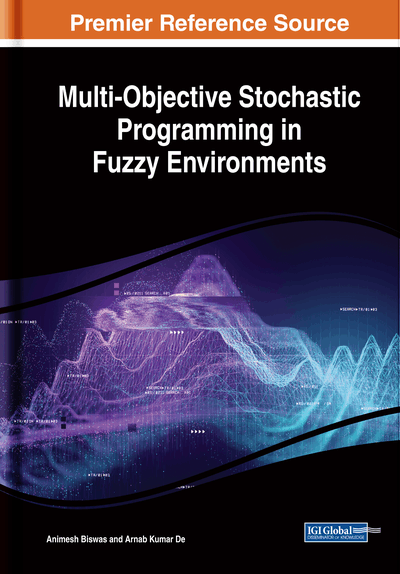# Fully Fuzzified Multi-Objective Stochastic Programming

DOI: 10.4018/978-1-5225-8301-1.ch006
Available
\$33.75
List Price: \$37.50
10% Discount:-\$3.75
TOTAL SAVINGS: \$3.75

## Abstract

This chapter develops a methodology for solving fully fuzzified multi-objective chance constrained programming (CCP) problems with fuzzy random variables (FRVs) as parameters. In the preceding chapters, it is assumed that the parameters of the multi-objective programming models are uncertain, and these uncertain parameters are expressed through fuzzy numbers (FNs) and FRVs. However, in practical situations, it is also observed that not only the parameters but also the variables of the multi-objective programming problems are uncertain. From that view point, the methodology for solving fully fuzzified multi-objective stochastic programming problems are presented in this chapter. At first the fuzzy probabilistic constraints are modified into fuzzy constraints. Using the defuzzification method of FNs, the different fuzzy parameters and fuzzy variables in the constraints are converted into crisp equivalent parameters and crisp variables. In this chapter, the parameters of the objectives are considered as either symmetric trapezoidal FNs or FRVs whose mean and variances are taken as symmetric trapezoidal FNs. If the parameters of the objectives are FRVs, then expectation model and variance model of the objective is used to find an equivalent form of the objectives whose parameters are only FNs. The ranking function of FNs is then applied to the objectives to convert them into crisp objectives. Then each objective is solved independently under the modified system constraints to construct the membership goals of each objective. Finally, weighted fuzzy goal programming (FGP) model is applied to achieve the most satisfactory solution for the overall benefit of the organization. Two illustrative numerical examples are given to demonstrate the efficiency of the proposed methodology and to compare the solution obtained by the developed methodology with the pre-defined techniques.
Chapter Preview
Top

## 6.1 Multi-Objective Fully Fuzzified Stochastic Programming

The classical multi-objective programming is used to find an optimal solution in many real life decision making problems, especially, in engineering and management. However, in a real world environment the value of many parameters are not precisely known. Usually the values of these parameters are estimated by experts and it cannot be assumed that the knowledge of experts is precise enough. Bellman and Zadeh (1970) proposed the concept of decision making in fuzzy environments. Tanaka et al. (1973) adopted these concepts to solve mathematical programming problems. Zimmermann (1978) first proposed the formulation of fuzzy linear programming problem (FLPP). Delgado et al. (1989) proposed a general model for the FLPPs in which constraints are fuzzy inequality and the parameters of the constraints are FNs but the parameters of the objective functions are crisp. Afterwards, a plenty number of researchers exhibited their interests to solve the FLPPs (Hop, 1991; Liu, 2001; Chiang, 2001). The FLPP can also be solved by multi-objective optimization method (Zhang et al., 2003), penalty method (Jamison and Lodwick, 2001), semi-infinite programming method (Leon and Vercher, 2004). Ganesan and veeramani (2006) proposed a method for solving FLPPs without converting them to crisp linear programming problems (LPP). In recent years, several kinds of fully fuzzified LPPs (FFLPPs) are appeared in the literature. The FLPP in which all the parameters as well as variables are FNs is known as FFLPP. Buckley and Feuring (2000) introduced a method to find the solution for FFLPP by changing the objective function into the multi-objective FLPPs (MOFLPPs). Dehghan et al. (2006) used a computational method to find the exact solution of an FFLPP. Allahviranloo et al. (2008) proposed a method for solving FFLPP by using a kind of defuzzification method. Kaur and Kumar (2011) proposed a new method for solving FFLPP in which some or all the parameters are represented by unrestricted L-R FNs. Hosseinzadeh and Edalatpanah (2016) proposed a methodology for solving FFLPP by using the lexicography method. Das et al. (2017) developed a mathematical model for solving FFLPP with trapezoidal FNs. Recently, Sharma and Agarwal (2018) developed methodology for solving MOFLPP using nearest interval approximation of FNs and interval programming.

Also, as described in preceding chapters, the decision makers (DMs) often face another type of uncertainty known as probabilistic uncertainty. CCP methodology is one the most useful techniques for dealing with such type of probabilistic uncertainties. The CCP model was first proposed by Charnes and Cooper (1962, 1963). Further the concept of CCP technique was extended by several researchers (Kataoka, 1963; Geoffrion, 1967) for solving different type of problems. With this improvement in computational resources and scientific computing techniques many complex optimization models can now be solved proficiently.

## Complete Chapter List

Search this Book:
Reset